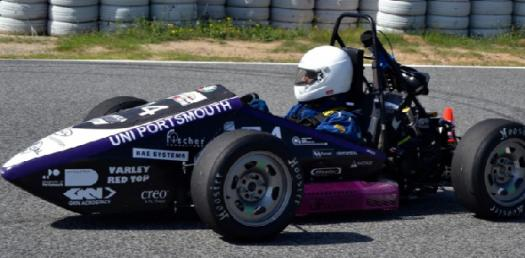Fs Austria Quiz 2

14 Questions | Total Attempts: 331Settings• 1.
What is the speed of the vehicle that needs to be achieved for the acceleration event?
• A.

35 kmph

• B.

50 kmph

• C.

40kmph

• D.

35 mil/hr

• 2.
What is the color and power wattage of the brake light used in the vehicle?
• A.

Red,10 watts

• B.

Yellow, 15 watts

• C.

Red, 15 watts

• D.

Yellow, 10 watts

• 3.
Which of the following is not affected by Center of Gravity of the vehicle?
• A.

Rolling and Pitching Moments

• B.

Downforce

• C.

Weight Distribution

• D.

• 4.
Which of the following is incorrect  for the Endurance Event ?
• A.

Elapsed time will begin when Driver A is ready to enter the vehicle for the coarse

• B.

Elapsed time will begin when Driver A enters the course and crosses the timing line

• C.

Elapsed time will begin when Driver A is inside the vehicle ready to start without starting the vehicle

• D.

Elapsed time will begin when Driver A is inside the vehicle and ready to start with the ignition on.

• 5.
Which of the following is not a positive locking mechanism ?
• A.

Safety Wiring

• B.

• C.

Nylon patches

• D.

Lock nuts

• 6.
What is the root cause of the problem with this steering system ?
• A.

Steering wheel mounting

• B.

Steering column support mounting

• C.

Steering rack mounting

• D.

Steering wheel too small

• 7.
Where can the Peltier effect be used ?
• A.

To Study Fluid Property

• B.

Thermocouple

• C.

Vehicle's Speed sensor

• D.

Current Sensor

• 8.
Which department is a problem by looking at this picture ?
• A.

Steering

• B.

Design

• C.

Wheel Assembly

• D.

Brakes

• 9.
It is given that x + 45= h/(tan30) and x = h/(tan50). Find the value of h.
• A.

42.26

• B.

63.19

• C.

50.39

• D.

28.69

• 10.
All the cost in the cost report will be submitted under :
• A.

Tool Cost

• B.

Unit Cost

• C.

Process Cost

• D.

Variable Cost

• 11.
In a single cylinder 4 stroke engine , the rating is 1500 rpm. Calculate the engine working in terms of "sec/stroke" :
• A.

50 sec/stroke

• B.

0.02 sec/stroke

• C.

64.25 sec/stroke

• D.

0.125 sec/stroke

• 12.
• A.

Output impedance and input impedance be high

• B.

Output impedance must be low and input impedance must be high

• C.

Output impedance must be high and input impedance must be low

• D.

Output impedance and input impedance must be low

• 13.
The internal RAM memory of 8051 is:
• A.

32bytes

• B.

64bytes

• C.

1024bits

• D.

2048bits

• 14.
Calculate the work done for a 4 cylinder, 4 stroke engine running at 1500 rpm with a cross section area of 1600 mm-square and the stroke length of 460 cm. If the mean effective pressure(MEP) is  150 N/m-square. Calculate the Work Done by the engine
• A.

52992

• B.

26496

• C.

57482

• D.

23845

Related TopicsBack to top